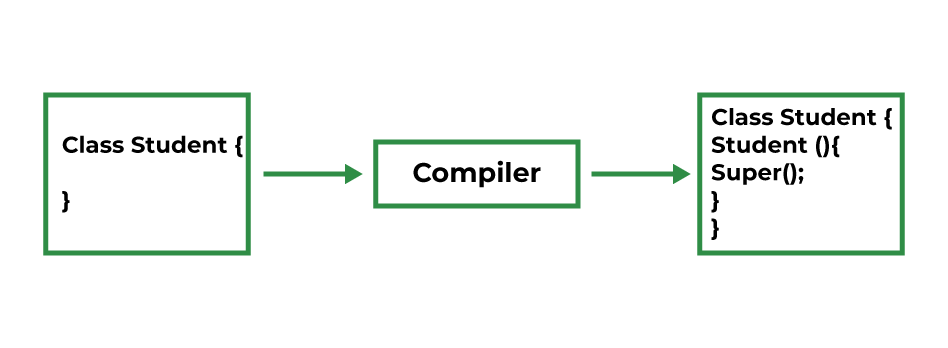Open in App
Not now

# Super Keyword in Java

• Difficulty Level : Easy
• Last Updated : 17 Oct, 2022

The super keyword in java is a reference variable that is used to refer to parent class objects. An understanding of Inheritance and Polymorphism is needed in order to understand the super keyword. The keyword “super” came into the picture with the concept of Inheritance. It is majorly used in the following contexts:

• Use of super with variables
• Use of super with methods
• Use of super with constructors

## 1. Use of super with variables

This scenario occurs when a derived class and base class has the same data members. In that case, there is a possibility of ambiguity for the JVM. We can understand it more clearly using this code snippet:

## Java

 `// Java code to show use of super keyword with variables` `// Base class vehicle` `class` `Vehicle {` `    ``int` `maxSpeed = ``120``;` `}`   `// sub class Car extending vehicle` `class` `Car ``extends` `Vehicle {` `    ``int` `maxSpeed = ``180``;`   `    ``void` `display()` `    ``{` `        ``// print maxSpeed of base class (vehicle)` `        ``System.out.println(``"Maximum Speed: "` `                           ``+ ``super``.maxSpeed);` `    ``}` `}`   `// Driver Program` `class` `Test {` `    ``public` `static` `void` `main(String[] args)` `    ``{` `        ``Car small = ``new` `Car();` `        ``small.display();` `    ``}` `}`

Output

`Maximum Speed: 120`

In the above example, both base class and subclass have a member maxSpeed. We could access maxSpeed of base class in subclass using super keyword.

## 2. Use of super with methods

This is used when we want to call the parent class method. So whenever a parent and child class have the same-named methods then to resolve ambiguity we use the super keyword. This code snippet helps to understand the said usage of the super keyword.

## Java

 `// Java program to show use of super with methods`   `// superclass Person` `class` `Person {` `    ``void` `message()` `    ``{` `        ``System.out.println(``"This is person class\n"``);` `    ``}` `}` `// Subclass Student` `class` `Student ``extends` `Person {` `    ``void` `message()` `    ``{` `        ``System.out.println(``"This is student class"``);` `    ``}` `    ``// Note that display() is` `    ``// only in Student class` `    ``void` `display()` `    ``{` `        ``// will invoke or call current` `        ``// class message() method` `        ``message();`   `        ``// will invoke or call parent` `        ``// class message() method` `        ``super``.message();` `    ``}` `}` `// Driver Program` `class` `Test {` `    ``public` `static` `void` `main(String args[])` `    ``{` `        ``Student s = ``new` `Student();`   `        ``// calling display() of Student` `        ``s.display();` `    ``}` `}`

Output

```This is student class
This is person class```

In the above example, we have seen that if we only call method message() then, the current class message() is invoked but with the use of the super keyword, message() of superclass could also be invoked.

## 3. Use of super with constructors

The super keyword can also be used to access the parent class constructor. One more important thing is that ‘super’ can call both parametric as well as non-parametric constructors depending upon the situation. Following is the code snippet to explain the above concept:

## Java

 `// Java Code to show use of ` `// super keyword with constructor`   `// superclass Person` `class` `Person {` `    ``Person()` `    ``{` `        ``System.out.println(``"Person class Constructor"``);` `    ``}` `}`   `// subclass Student extending the Person class` `class` `Student ``extends` `Person {` `    ``Student()` `    ``{` `        ``// invoke or call parent class constructor` `        ``super``();`   `        ``System.out.println(``"Student class Constructor"``);` `    ``}` `}`   `// Driver Program` `class` `Test {` `    ``public` `static` `void` `main(String[] args)` `    ``{` `        ``Student s = ``new` `Student();` `    ``}` `}`

Output

```Person class Constructor
Student class Constructor```

In the above example, we have called the superclass constructor using the keyword ‘super’ via subclass constructor.

### Important Points to Remember while using Super Keyword

• Call to super() must be the first statement in the Derived(Student) Class constructor because if you think about it, it makes sense that the superclass has no knowledge of any subclass, so any initialization it needs to perform is separate from and possibly prerequisite to any initialization performed by the subclass. Therefore, it needs to complete its execution first.
• If a constructor does not explicitly invoke a superclass constructor, the Java compiler automatically inserts a call to the no-argument constructor of the superclass. If the superclass does not have a no-argument constructor, you will get a compile-time error. The object does have such a constructor, so if the Object is the only superclass, there is no problem.• If a subclass constructor invokes a constructor of its superclass, either explicitly or implicitly, you might think that a whole chain of constructors is called, all the way back to the constructor of Object. This, in fact, is the case. It is called constructor chaining.